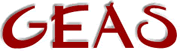[ < ] [ > ] [ << ] [ Up ] [ >> ] [Top] [Contents] [Index] [ ? ]

#### 1.2.7.2 Arithmetic operators

1. E1 + E2 The expressions are evaluated and the results added to each other.

You can add integers, floats, strings, arrays and mappings. Strings, arrays and mappings are simply concatenated - pasted together to the end of the first argument.

It's also possible to add integers to strings, they will then be converted to strings and pasted to the end of the string.

2. E1 - E2 E2 is subtracted from E1.

You can subtract integers, floats and any type from arrays of the same type. For arrays the item, if it exists in the array it is subtracted from, is removed from the array. If it doesn't exist in the array, the array is returned intact.

3. E1 * E2 E1 is multiplied by E2.

This only works on integers and floats.

4. E1 / E2 E1 is divided by E2.

This only works on integers and floats.

5. E1 % E2 The remainder of the expression 'E1 / E2' is returned.

This only works with integers.

 ```'14 % 3' will yield 2 as the remainder. '14 / 3' will be 4, and 4 * 3 + 2 = 14 as a small check. ```

6. -E Return E with reversed sign.

This only works on integers and floats.

7. E++, ++E The expression 'E' is incremented by one. If the operator is in front of the expression, the incrememntation is done before the expression is used, otherwise afterwards.

 ```'a = 3; b = ++a;' will yield the result 'a = 4, b = 4', while 'a = 3; b = a++;' will yield the result 'a = 4, b = 3'. ```

This only works on integers.

8. E--, --E The expression 'E' is decremented by one. If the operator is in front of the expression, the decrementation is done before the expression is used, otherwise afterwards.

 ```'a = 3; b = --a;' will yield the result 'a = 2, b = 2', while 'a = 3; b = a--;' will yield the result 'a = 2, b = 3'. ```

This only works on integers.

 [ < ] [ > ] [ << ] [ Up ] [ >> ] [Top] [Contents] [Index] [ ? ]

This document was generated by Ronny Wikh on July, 8 2003 using texi2html